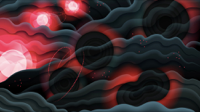Cococubed.com Black Hole Mass Spectrum

Home

Astronomy research
Software Infrastructure:
MESA
FLASH-X
STARLIB
MESA-Web
starkiller-astro
My instruments
White dwarf pulsations:
12C(α,γ) & overshooting
Probe of 12C(α,γ)16O
Impact of 22Ne
Impact of ν cooling
Variable white dwarfs
MC reaction rates
Micronovae
Novae
White dwarf supernova:
Stable nickel production
Remnant metallicities
Colliding white dwarfs
Merging white dwarfs
Ignition conditions
Metallicity effects
Central density effects
Detonation density
Tracer particle burning
Subsonic burning fronts
Supersonic fronts
W7 profiles
Massive stars:
Pop III with HST/JWST
Rotating progenitors
3D evolution to collapse
MC reaction rates
Pre-SN variations
Massive star supernova:
26Al & 60Fe
44Ti, 60Co & 56Ni
SN 1987A light curve
Constraints on Ni/Fe
An r-process
Effects of 12C +12C
Neutron Stars and Black Holes:
Black Hole spectrum
Mass Gap with LVK
Compact object IMF
He burn neutron stars
Neutrino Emission:
Neutrino emission from stars
Identifying the Pre-SN
Neutrino HR diagram
Pre-SN Beta Processes
Pre-SN neutrinos
Stars:
Hypatia catalog
SAGB stars
Nugrid Yields I
He shell convection
BBFH at 40 years
γ-rays within 100 Mpc
Iron Pseudocarbynes
Pre-Solar Grains:
C-rich presolar grains
SiC Type U/C grains
Grains from massive stars
Placing the Sun
SiC Presolar grains
Chemical Evolution:
Zone models H to Zn
Mixing ejecta
Thermodynamics & Networks
Skye EOS
Helm EOS
Five EOSs
Equations of State
12C(α,γ)16O Rate
Proton-rich NSE
Reaction networks
Bayesian reaction rates
Verification Problems:
Validating an astro code
Su-Olson
Cog8
RMTV
Sedov
Noh
Software instruments
Presentations
Illustrations
Public Outreach
Education materials
2023 ASU Solar Systems Astronomy
2023 ASU Energy in Everyday Life

AAS Journals
2023 AAS Peer Review Workshops
2023 MESA VI
2023 MESA Marketplace
2023 MESA Classroom
2023 Neutrino Emission from Stars
2023 White Dwarfs & 12C(α,γ)16O
2022 Earendel, A Highly Magnified Star
2022 Black Hole Mass Spectrum
2022 MESA in Don't Look Up
2021 Bill Paxton, Tinsley Prize

Contact: F.X.Timmes
my one page vitae,
full vitae,
research statement, and
teaching statement.
Resolving The Peak Of The Black Hole Mass Spectrum (2022)

Gravitational wave (GW) detections of binary black hole (BH) mergers have begun to sample the cosmic BH mass distribution. The evolution of single stellar cores predicts a gap in the BH mass distribution due to pair-instability supernova (PISN). Determining the upper and lower edges of the BH mass gap can be useful for interpreting GW detections from merging BHs. In this article We use MESA to evolve single, non-rotating, massive helium cores with a metallicity of $Z = 10^{-5}$ until they either collapse to form a BH or explode as a PISN without leaving a compact remnant. We calculate the boundaries of the lower BH mass gap for S-factors in the range S(300 keV) = (77,203) keV b, corresponding to the $\pm 3\sigma$ uncertainty in our high resolution tabulated $^{12}$C($\alpha$,$\gamma$)$^{16}$O reaction rate probability distribution function. We extensively test the temporal and mass resolution to resolve the theoretical peak of the BH mass spectrum across the BH mass gap. We explore the convergence with respect to convective mixing and nuclear burning, finding that significant time resolution is needed to achieve convergence. We also test adopting a minimum diffusion coefficient to help lower resolution models reach convergence. We establish a new lower edge of the upper mass gap as M$_{\rm lower}$ $\simeq$ 60$^{+32}_{-14}$ M$_{\odot}$ from the $\pm 3\sigma$ uncertainty in the $^{12}$C($\alpha$,$\gamma$)$^{16}$O rate. We explore the effect of a larger 3-$\alpha$ rate on the lower edge of the upper mass gap, finding M$_{\rm lower}$ $\simeq$ 69$^{+34}_{-18}$ M$_{\odot}$. We compare our results with BHs reported in the Gravitational-Wave Transient Catalog.

Observing Intermediate-mass Black Holes and the Upper Stellar-mass gap with LIGO and Virgo (2022)

In this article we probe the mass function of intermediate-mass black holes (IMBHs) wherein we also include BHs in the upper mass gap ∼60 - 130 M$_{\odot}$. Employing the projected sensitivity of the upcoming LIGO and Virgo fourth observing (O4) run, we perform Bayesian analysis on quasi-circular non-precessing, spinning IMBH binaries (IMBHBs) with total masses 50 - 500 M$_\odot$, mass ratios 1.25, 4, and 10, and dimensionless spins up to 0.95, and estimate the precision with which the source-frame parameters can be measured. We find that, at 2$\sigma$, the mass of the heavier component of IMBHBs can be constrained with an uncertainty of ∼10 - 40% at a signal-to-noise ratio of 20. Focusing on the stellar-mass gap with new tabulations of the $^{12}$C($\alpha$,$\gamma$)$^{16}$O reaction rate and its uncertanties, we evolve massive helium core stars using MESA, to establish the lower and upper edge of the mass gap as ∼59$^{+34}_{-13}$ M$_{\odot}$ and ∼139$^{+30}_{-14}$ M$_{\odot}$ respectively, where the error bars give the mass range that follows from the ±3$\sigma$ uncertainty in the $^{12}$C($\alpha$,$\gamma$)$^{16}$O nuclear reaction rate. We find that high resolution of the tabulated reaction rate and fine temporal resolution are necessary to resolve the peak of the BH mass spectrum. We then study IMBHBs with components lying in the mass gap and show that the O4 run will be able to robustly identify most such systems. Finally, we re-analyse GW190521 with a state-of-the-art aligned-spin waveform model, finding that the primary mass lies in the mass gap with 90% credibility.